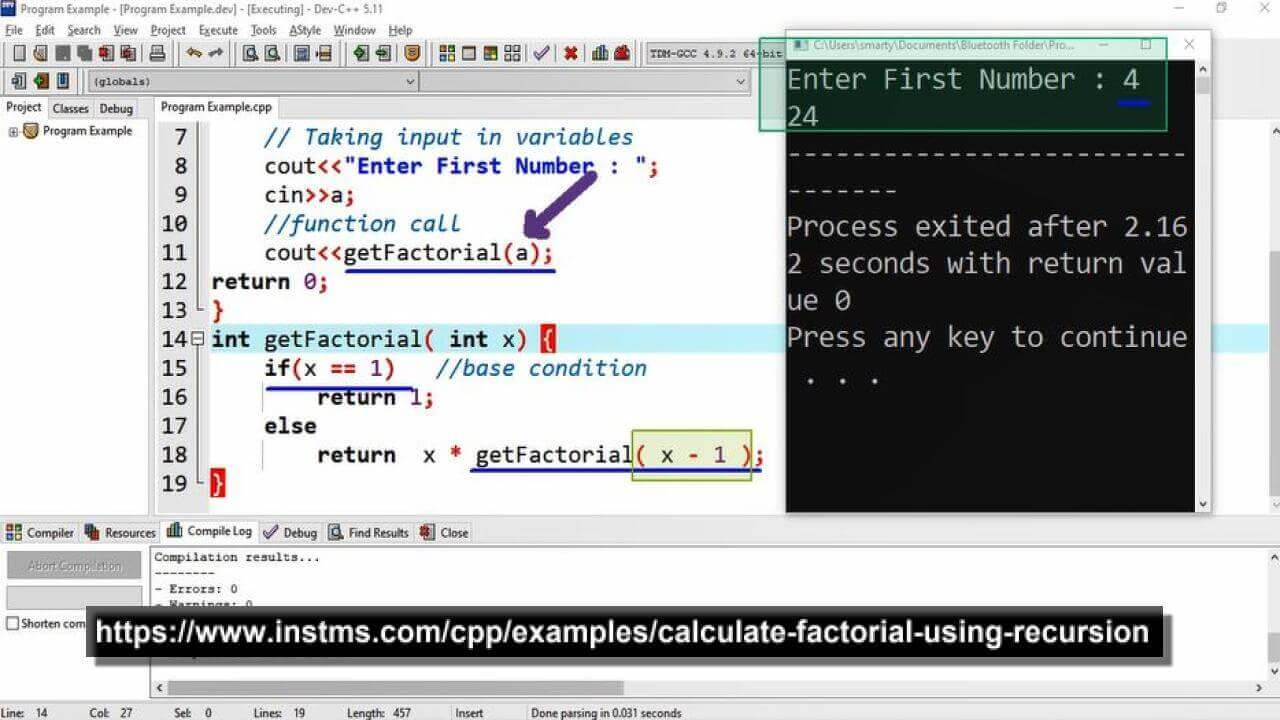# Calculate Factorial Using Recursion in CPP

## Source Code

#include <iostream>

using namespace std;

int getFactorial( int );

int main(int argc, char** argv) {

int a;  // variable declaration for input

// Taking input in variables
cout<<"Enter First Number : ";
cin>>a;
//function call
cout<<getFactorial(a);

return 0;
}

int getFactorial( int x) {

if(x == 1)   //base condition
return 1;
else
return  x * getFactorial( x - 1 );  // recursive call
}

## Output## Working

This program use recursion method for calculation of factorial. Before this we have used Loops (factorial using while loop) for same results. In this program a function named as getFactorial is written and called recursively for factoial calculation.